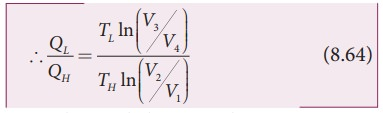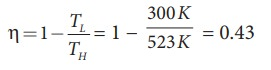Home | | Physics 11th std | Efficiency of a Carnot engine

# Efficiency of a Carnot engine

Efficiency is defined as the ratio of work done by the working substance in one cycle to the amount of heat extracted from the source.

Efficiency of a Carnot engine

Efficiency is defined as the ratio of work done by the working substance in one cycle to the amount of heat extracted from the source.From the first law of thermodynamics,

W = QH ŌłÆ QLHere we omit the negative sign. Since we are interested in only the amount of heat (QL) ejected into the sink, we haveBy applying adiabatic conditions, we get,

TH V2╬│ ŌłÆ1 =TLV3╬│ŌłÆ1

TH V1╬│ ŌłÆ1 =TLV4╬│ŌłÆ1

By dividing the above two equations, we getNote : TL and TH  should be expressed in Kelvin scale.

## Important results:

1. ╬Ę is always less than 1 because TL is less than TH. This implies the efficiency cannot be 100%. It can be 1 or 100% only when TL = 0K (absolute zero of temperature) which is impossible to attain practically.

2. The efficiency of the CarnotŌĆÖs engine is independent of the working substance. It depends only on the temperatures of the source and the sink. The greater the difference between the two temperatures, higher the efficiency.

3. When TH=TL the efficiency ╬Ę =0. No engine can work having source and sink at the same temperature.

4. The entire process is reversible in the Carnot engine cycle. So Carnot engine is itself a reversible engine and has maximum efficiency. But all practical heat engines like diesel engine, petrol engine and steam engine have cycles which are not perfectly reversible. So their efficiency is always less than the Carnot efficiency. This can be stated in the form of the Carnot theorem. It is stated as follows ŌĆśBetween two constant temperatures reservoirs, only Carnot engine can have maximum efficiency. All real heat engines will have efficiency less than the Carnot engineŌĆÖ

### EXAMPLE 8.25

a) A steam engine boiler is maintained at 250┬░C and water is converted into steam. This steam is used to do work and heat is ejected to the surrounding air at temperature 300K. Calculate the maximum efficiency it can have?

### Solution

The steam engine is not a Carnot engine, because all the process involved in the steam engine are not perfectly reversible. But we can calculate the maximum possible efficiency of the steam engine by considering it as a Carnot engine.The steam engine can have maximum possible 43% of efficiency, implying this steam engine can convert 43% of input heat into useful work and remaining 57% is ejected as heat. In practice the efficiency is even less than 43%.

### EXAMPLE 8.26

There are two Carnot engines A and B operating in two different temperature regions. For Engine A the temperatures of the two reservoirs are 150┬░C and 100┬░C. For engine B the temperatures of the reservoirs are 350┬░C and 300┬░C. Which engine has lesser efficiency?

### Solution

The efficiency for engine A = 1 ŌłÆ 373/423 = 0.11. Engine A has 11% efficiency

The efficiency for engine B = 1 - 573/623 = 0.08

Engine B has only 8% efficiency.

Even though the differences between the temperature of hot and cold reservoirs in both engines is same, the efficiency is not same. The efficiency depends on the ratio of the two temperature and not on the difference in the temperature. The engine which operates in lower temperature has highest efficiency.

Tags : Thermodynamics , 11th Physics : UNIT 8 : Heat and Thermodynamics
Study Material, Lecturing Notes, Assignment, Reference, Wiki description explanation, brief detail
11th Physics : UNIT 8 : Heat and Thermodynamics : Efficiency of a Carnot engine | Thermodynamics